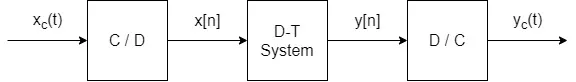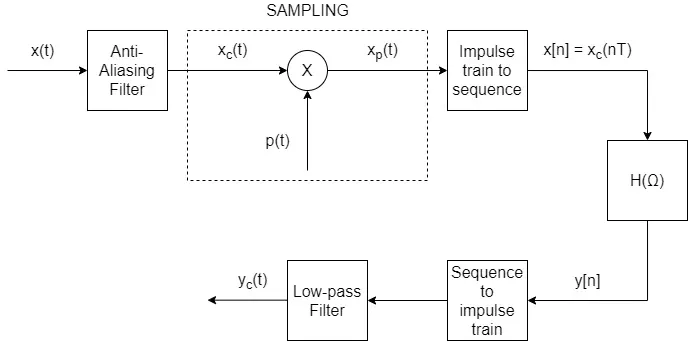# Mathematics - Signals And Systems - Processing Continuous-Time Signals as Discrete-Time Signals[Image1]

## Introduction

Hey it's a me again @drifter1!

Today we continue with my mathematics series about Signals and Systems in order to cover Processing Continuous-Time Signals as Discrete-Time Signals.

So, without further ado, let's get straight into it!

## Continuous-Time to Discrete-Time Conversion (and vise versa)

Maybe the most important application of sampling is its role in processing continuous-time signals using discrete-time systems. Continuous-Time signals have to be band-limited in order for the conversion to work. If they are already band-limited they can easily be sampled, and those samples are then converted to some kind of discrete-time representation. On the other hand, if they are not band-limited that are first processed with an anti-aliasing filter (which band-limits them) and then sampled.The resulting discrete-time representation of the continuous-time signals can be processed by discrete-time systems and when the whole procedure is finished desampled/reconstructed into continuous-time signals. This is done using preferably band-limited interpolation, which allows converting the sequence to an (im)pulse train. This (im)pulse train is then processed through a low-pass filter, which in turn gives the final continuous-time signal. Of course the exact sampling period, the periodicy of the to-be-sampled signal, and much much more that we already discussed throughout the series, also affects the outcome of this whole procedure.

## Discrete-Time Processing

Whilst converting the impulse train of samples to a sequence of samples, we basically normalize the time-axis. Recalling the properties of the Fourier Transform, its easy to conclude that the discrete-time Fourier transform of the sequence of samples gives the same result as the continuous-time Fourier transform of the impulse train, if the frequency-axis is normalized in that case. In other words, processing the continuous-time signal as an discrete-time signal is not violating the Fourier transform principles, and the result after the sampler is the same that we would get processing the continuous-time signal directly.

To get even more specific, while converting the result back to a continuous-time signal, we basically "un-normalize" the frequency-axis. So, the overall system which processes the discrete-time representation is equivalent to a continuous-time filter with a frequency response that is the same as the frequency resposne of the discrete-tiem filter if linear scaled on the frequency-axis. The cut-off frequency in this case depends on the sampling frequency, and so it can be varied by varying the sampling frequency.

## Complete Block Diagram## RESOURCES:

### Images

1.Block diagrams and other visualizations were made using draw.io and GeoGebra

## Previous articles of the series

### Filtering, Sampling, Modulation, Interpolation

• Filtering → Convolution Property, Ideal Filters, Series R-C Circuit and Moving Average Filter Approximations
• Continuous-Time Modulation → Getting into Modulation, AM and FM, Demodulation
• Discrete-Time Modulation → Applications, Carriers, Modulation/Demodulation, Time-Division Multiplexing
• Sampling → Sampling Theorem, Sampling, Reconstruction and Aliasing
• Interpolation → Reconstruction Procedure, Interpolation (Band-limited, Zero-order hold, First-order hold)

## Final words | Next up

And this is actually it for today's post!

Next time we will get into discrete-time sampling...

See Ya!Keep on drifting!

H2
H3
H4
3 columns
2 columns
1 column
Join the conversation now